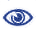# Prague Stringology Conference 2011

Computing Longest Common Substring/Subsequence of Non-linear Texts

 Abstract: A non-linear text is a directed graph where each vertex is labeled with a string. In this paper, we introduce the longest common substring/subsequence problems on non-linear texts. Firstly, we present an algorithm to compute the longest common substring of non-linear texts G1 and G2 in O(|E1||E2|) time and O(|V1||V2|) space, when at least one of G1 and G2 is acyclic. Here, Vi and Ei are the sets of vertices and arcs of input non-linear text Gi, respectively, for 1 ≤ i ≤ 2. Secondly, we present algorithms to compute the longest common subsequence of G1 and G2 in O(|E1||E2|) time and O(|V1||V2|) space, when both G1 and G2 are acyclic, and in O(|E1||E2| + |V1||V2| log |Σ|) time and O(|V1||V2|) space if G1 and/or G2 are cyclic, where, Σ denotes the alphabet.

 Download paper:PostScript PDF BibTeX reference
 Download presentation: